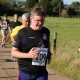## A (partial) answer to my Goedelian conundrum?

Last week I puzzled over what seemed to me to be the hand waiving dismissal, by both Alan Turing and Douglas Hofstadter of what I saw as the problem of humans being able to write true statements that the formal systems employed by computers could not determine – the problem thrown up by Goedel’s Incompleteness Theorems.

Well, Douglas Hofstadter has now come to his own (partial) rescue as I continue to read on through Godel, Escher, Bach– as he describes Tarski’s Theorem, which essentially states that humans cannot determine all such statements either (unless we posit the Church-Turing thesis is wrong, of course and there is some inner human computational magic we have yet to describe).

I am now going to quickly run through Hofstadter’s exposition – it might not mean too much to those of you not familiar with GEB, but if so and if you are interested in computation (and genetics and music) and you want to improve your mind this summer you could always think about buying the book. I don’t promise it’s an easy read, the style can vary from the nerdy to the deeply frustrating, but it is still a rewarding read.

So here goes:

We imagine we have a procedure that can determine the truth of a number theoretical statement, i.e.:$TRUE\{a\}$ can tell us whether the number theoretical statement with Goedel number a is true.

So now we posit,$T$ with Goedel number$t$:$\exists a:<\sim TRUE\{a\} \wedge ARITHMOQUINE\{a^{\prime\prime},a\}>$

Now, of you have read GEB you will know that to “arithmoquine” a number theoretical statement is to replace the free variable – in this case$a^{\prime\prime}$, with the Goedel number for the statement itself…$\exists a:<\sim TRUE\{a\} \wedge ARITHMOQUINE\{SSS \ldots SSS0,a\}>$

Which we can state as “the arithmoquinification of$t$ is the Goedel number of a false statement”.

But the arithmoquinification of$t$ is$T$‘s own number, so this statement is the equivalent of saying “this statement is false”: just another version of the famous Epimenides Paradox, but one that is decidedly not hand waving in form: it’s about natural numbers.

The outcome is that$TRUE\{a\}$ cannot exist without our whole idea of natural numbers collapsing and we are forced to conclude there is no formal way of deciding what is a true statement of theoretical number theory using only theoretical number theory – and so humans are no better off than computers in this regard: we use concepts from without the formal theory to establish truth and we could surely program our computers to do the same. Turing’s “imitation game” conception of intelligent machines thus survives.

At least I think so!

2.